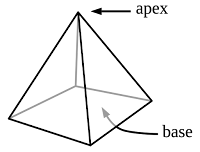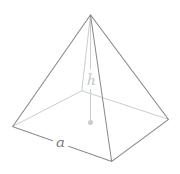## Detailed Concepts and formulae of Pyramid and tetrahedron for SSC CGL Exam

A Pyramid is a three-dimensional polyhedron of which one face is a polygon of any number of sides, and the other faces are triangles with a common vertex.

A pyramid with an n-sided base will have n + 1 vertices, n + 1 faces, and 2n edges.Right Pyramid: A right pyramid has its apex directly above the centroid of its base.

Regular Pyramid: A regular pyramid has a regular polygon base.

Generic Formulas:

### Surface Area Of pyramid = 1/2 × Perimeter of base  × Slant Length

(only when all side faces are the same)

Surface Area = Base Area + Lateral Area
(When side faces are different)

### Regular Right Pyramid with Square base (pentahedron):

Square pyramid is a pyramid having a Square base and the other faces are all triangles.

There are (4+1) faces, 5 vertices and 8 edges in a pentahedron.Formulas:

V = 1/3 Bhwhere, e is the edge length, s is the slant height, h is the height, A is the Surface area, AL is the lateral Surface area, B is the area of the square base and a is the length of a side of the base.

### Regular Right Pyramid with Triangular base (Tetrahedron):

triangular pyramid is a pyramid having a triangular base.

The tetrahedron is a triangular pyramid having congruent equilateral triangles for each of its faces.

There are 4 faces, 4 vertices and 6 edges in a tetrahedron.

h = (√2)/(√3) * (Side)

AL = (3√3)/4 * (Side)2

A = (4√3)/4 * (Side)2

V = (√2)/12 * (Side)3                (Since V= 1/3 Bh )

where, e is the edge length, s is the slant height, h is the height, AL is the lateral Surface area, A is the Surface area, V is Volume, B is the area of the base and a is the length of a side of the base.

|| Prism, || Cone, || Cylinder,  || Sphere,Hemispheres, ||
|| Pyramid ||

Concepts and formulae of Pyramid and Tetrahedron for SSC CGLReviewed by Admin on 1:54:00 PM Rating: 5